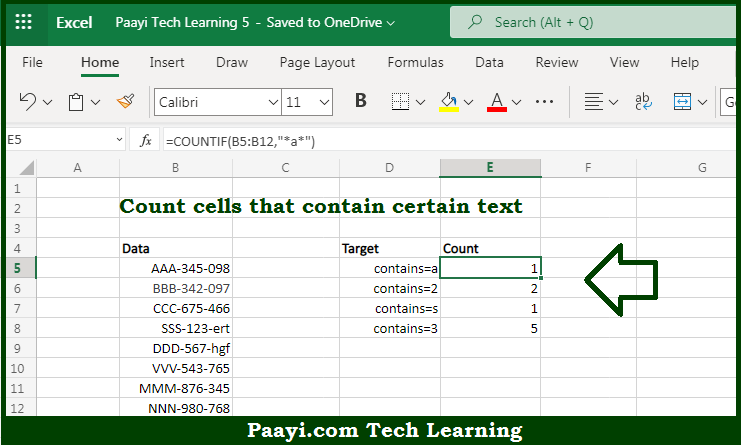# Learn How to COUNT Cells that Contain Specific Text in Microsoft Excel

Written by | 0 Comments | 349 Views

In this article, you will learn how to COUNT various things in Microsoft Excel using a single or combination of functions and its purpose in Microsoft Excel. You will also get to know how to count cells that contain specific text & generic formula

Count Cells that Contain Specific Text in Microsoft Excel

The main purpose of this formula is to count the number of cells that contain certain text. Here we will learn how to count the range of cells containing certain text in Microsoft Excel.  That implies, with the help of a formula based on the  COUNTIF function with a wildcard you can able to count the number of cells that contain the specified text. The generic form of the formula provided below "rng" represents a range of cells. So, with the help of this formula, you can able to count the number of cells that contain the specified text.

General Formula to COUNT Cells that Contain Specific Text

=COUNTIF(range,"*txt*")

The Explanation for the COUNT Cells that Contain Specific TextSo we know that with the help of the given formula above you can able to count the number of cells that contain certain text. Here we will learn how to count the range of cells containing certain text in Microsoft Excel. As we know that, the  COUNTIF function is used to count cells in a range that meets the criteria. It should be noted that the asterisk used in the formula means "match any number of characters, including zero", so this pattern will count any cell that contains "a" in any position, with or without other characters to the left or right. So, with the help of this formula, you can able to count cells that contain specific text.